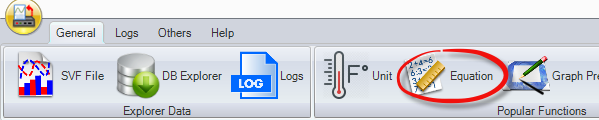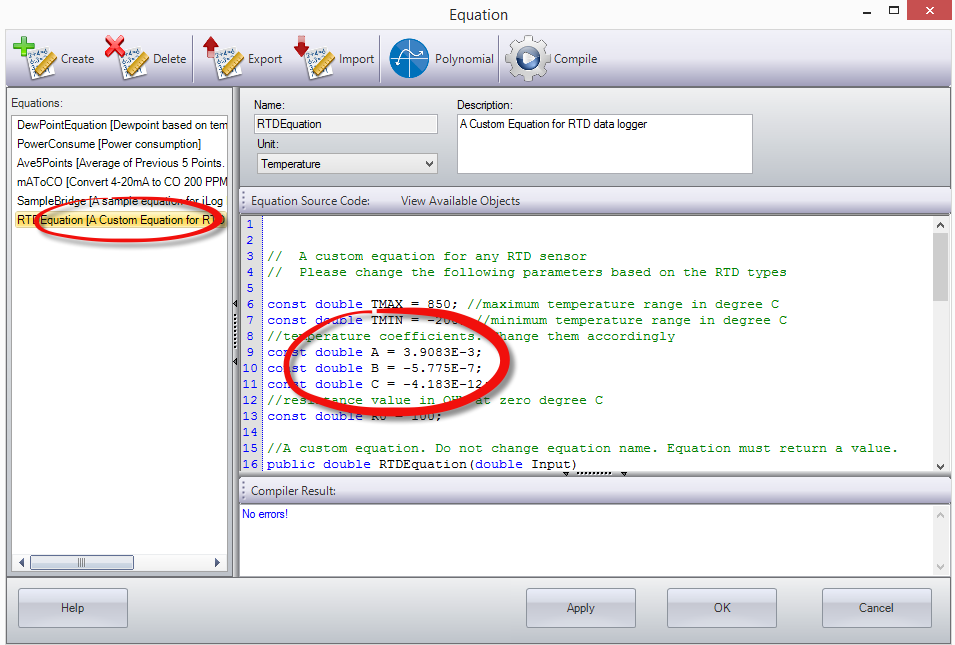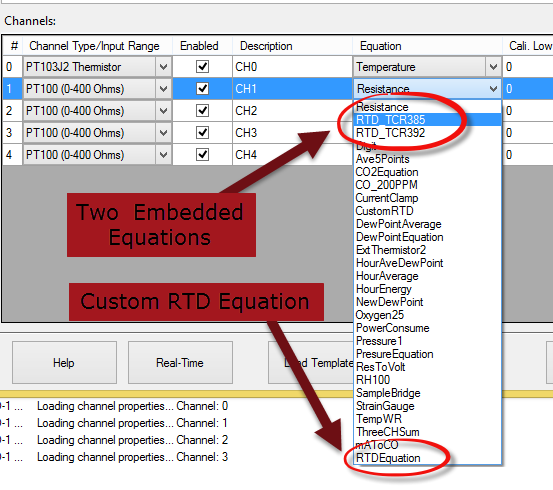# What Equation Should I use for a RTD Data Logger?

Microedge produces three models of RTD data loggers for different RTD sensors:

LRTD-1 for PT100
LRTD-2 for PT500
LRTD-3 for PT1000

Depending on the RTD sensor you are using you can use the following two embedded equations:

1. RTD_TCR385:This equation is for Temperature Coefficient of Resistance (TCR) 0.00385Ω/Ω/°C .
The temperature coefficients are:
A: 3.9083E-3
B: -5.775E-7
C: -4.183E-12
2. RTD_TCR392:This equation is for Temperature Coefficient of Resistance (TCR) 0.00392Ω/Ω/°C .
The temperature coefficients are:
A:  3.9848E-3
B: -5.87E-7
C: -4E-12

If your RTD’s temperature coefficients are different from either of the above, you should use a custom equation included in SiteView software.  The name of the equation is called “RTDEquation”.

You can open this equation and change those A, B and C values accordingly:

1. Click “Equation” toolbar menu item:2. Choose RTDEquation from the list and change parameters. Click “Compile” to make sure no errors. Then click “OK” button to save the equation:If you are in the Configuration dialog of the RTD data logger, you can choose one of the above three equations from the pull-down list.Please refer to the product page or User’s Manual for details:

https://www.microedgeinstruments.com/lrtd.php